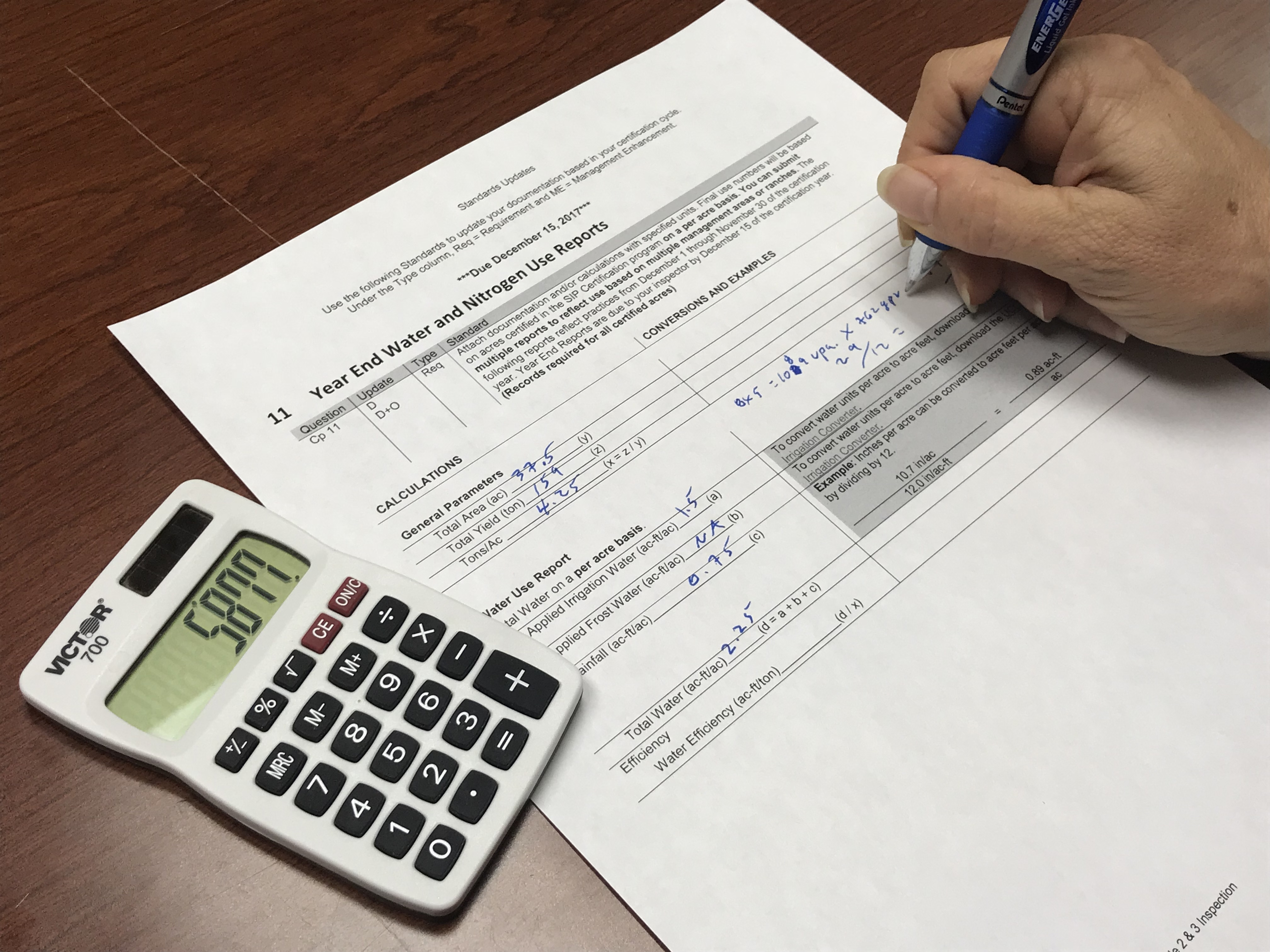Projects

# Liquid Fertilizer Calculations

## How Much Liquid Fertilizer to ApplyTo calculate how much liquid fertilizer you need to apply to deliver some number of pounds of Nitrogen to a field you need to know:
• The percentage of Nitrogen in the fertilizer (A)
• The weight per gallon of the fertilizer (B)
• How many pounds of Nitrogen you want to apply per acre (C)
• The total number of acres in the field (D)

### Calculations:

• Pounds of Nitrogen per gallon of fertilizer (E) = A x B
• Gallons of fertilizer required to deliver desired amount of Nitrogen to one acre (F) = C / E
• Number of gallons required to deliver desired amount of Nitrogen to entire field (G) = F x D

### Example

Desired application:
• 15 lbs. N (Nitrogen) per acre
• 20 acre field
Fertilizer, liquid:
• 10-10-10 formulation
• Product weighs 10.69 lbs. per gallon
• 10% of product is N
Calculations:
• 10.69 lbs./gal. x 0.10 = 1.069 lbs. of N per gallon
• 15 lbs. N/ac. ÷ 1.069 lbs. N/gallon = 14.03 gallons of product per acre required to deliver 15 lbs. N per acre
• 280.6 gallons of fertilizer required to fertilize entire field

### How Much Was Applied

To determine how much Nitrogen was actually applied to a field, the calculations are similar. You can use much of the information you already have about the fertilizer and the field.

The amount of fertilizer actually applied is often different than the target due to things like logistical issues.

In the example above, 280.6 gallons of fertilizer were required to hit the desired target of 15 lbs. of N. Applying 300 gallons of fertilizer instead of 280.6 gallons may have been cheaper or more efficient to deliver.

So how many pounds of Nitrogen were actually delivered per acre if 300 gallons of fertilizer were applied instead of 280.6?

• Total gallons of fertilizer (H)

• Gallons of fertilizer applied per acre (I) = H / D

• Pounds of Nitrogen applied per acre (J) = I x E

Using the example above, how many pounds of Nitrogen were applied per acre?

• 300 gallons of fertilizer applied to 20 acres = 15 gallons of fertilizer applied per acre

• 1.069 lbs. N/gallon x 15 gallons/acre = 16.03 lbs. N/acre applied

A Certified Crop Adviser (CCA) can make recommendations for how much to fertilize, when to fertilize, and how to deliver the fertilizer.

## Resources

PODCAST: Measuring Water and Nitrogen Use

SIP Certified Chapter 11 Standards- Year End Water and Nitrogen Use Reports (with calculations)

Find a Certified Crop Adviser

Compost Nitrogen Rate Calculator

Liquid Fertilizer Rate/Application Worksheet